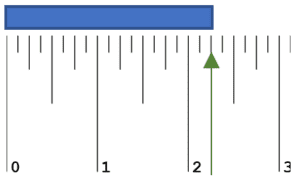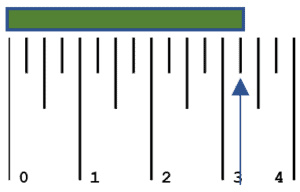## What Are Rulers?

Rulers are measuring tools used to determine the length, width, or thickness of an object or to draw straight lines. They are typically flat, rectangular, and made of various materials like plastic, metal, or wood. Rulers come in different sizes and units of measurement, with the most common being the imperial system (inches, feet) and the metric system (centimeters, millimeters).

There are several types of rulers, including:

Standard Ruler: This is the most common type of ruler, typically 12 inches (30 centimeters) long, with markings for inches and centimeters or millimeters. It’s used for measuring everyday objects and drawing straight lines.

Tape Measure: A flexible, retractable ruler made of metal or plastic, used for measuring larger distances or curved surfaces. They come in various lengths, with some measuring up to several meters or feet.

Architect’s Scale Ruler: A specialized ruler used by architects and engineers for drafting and measuring scaled drawings. It has multiple scales, allowing the user to convert measurements between different units or proportions.

Triangular Scale Ruler: A triangular ruler with six different scales, commonly used by architects, engineers, and designers for measuring and scaling drawings in various units or ratios.

T-square: A ruler with a perpendicular head, used in drafting and technical drawing for creating straight lines and right angles.

Protractor: A semicircular or circular measuring instrument with degree markings used for measuring angles, usually in degrees.

Rulers are essential tools in many professions, such as architecture, engineering, graphic design, and woodworking, as well as in everyday tasks that require precise measurement or drawing straight lines.

## How Do You Read a Ruler?

Reading a ruler is a basic skill that’s important for measuring lengths and distances. Rulers are typically marked with imperial (inches) or metric (centimeters and millimeters) units. Here’s a step-by-step guide on how to read a ruler:

Identify the Unit of Measurement: First, determine whether the ruler uses imperial (inches) or metric (centimeters and millimeters) units. Imperial units will usually have larger marks for inches and smaller marks for fractions of an inch, while metric units will have larger marks for centimeters and smaller marks for millimeters.

a. Locate the Zero Point: This is the end of the ruler where the measurements start.

b. Identify the Inch Marks: The longest lines on the ruler typically represent inches. They are usually numbered to make it easier to read.

c. Recognize the Fraction Marks: Between each inch mark, there will be smaller lines representing fractions of an inch. These are typically broken down into 1/2, 1/4, 1/8, and 1/16-inch marks. The longer the line, the larger the fraction.

d. Measure the Length: Line up the zero point with the start of the object you’re measuring. The distance between the zero point and the end of the object will be the length in inches and fractions of an inch.

Example:1. We located the zero point (left) and then we observe that ruler is broken into units of 1/8.
2. The object passes 2 Whole Units, we now need to determine the remaining fractional units.
3. The fractional portion of the measurement is 2 fractional units, since it is a 1/8 unit scale this means that fractional portion is 2/8.
4. When we combine the whole and fractional parts we get a final measurement of 2 2/8 cm.

a. Locate the Zero Point: This is the end of the ruler where the measurements start.

b. Identify the Centimeter Marks: The longer lines on the ruler typically represent centimeters. They are usually numbered to make it easier to read.

c. Recognize the Millimeter Marks: Between each centimeter mark, there will be nine smaller lines representing millimeters. There are 10 millimeters in a centimeter.

d. Measure the Length: Line up the zero point with the start of the object you’re measuring. The distance between the zero point and the end of the object will be the length in centimeters and millimeters.

Practice reading a ruler by measuring various objects and comparing your measurements with others to improve your accuracy and confidence.

Example:1. We located the zero point (left) and then we observe that ruler is broken into units of 1/4.
2. The object passes 3 Whole Units, we now need to determine the remaining fractional units.
3. The fractional portion of the measurement is 1 fractional unit, since it is a 1/4 unit scale this means that fractional portion is 1/4.
4. When we combine the whole and fractional parts we get a final measurement of 3 1/4 cm.

### How to Teach This Skill

Teaching kids how to use rulers is an important skill that helps them understand measurement, spatial relationships, and basic math concepts. Here are some steps and tips for teaching kids to use rulers:

Introduce Rulers

Show them a ruler and explain its purpose. Talk about the different types of rulers and the materials they can be made of (wood, plastic, metal). Teach them about the two main systems of measurement – imperial (inches and feet) and metric (centimeters and millimeters). Explain the difference between the systems and how to identify which one is being used on a particular ruler.

Break It Down Hand-on

Break down the process of reading a ruler step by step, as explained in a previous answer. Show them the inch or centimeter marks, and explain the smaller marks (fractions of an inch or millimeters). Give your child a ruler and have them measure various objects around the house, such as books, pencils, or toys. Encourage them to line up the zero point correctly and read the measurement accurately.

Turn the learning process into a game by having them guess the length of an object before measuring it or creating a scavenger hunt where they have to find objects of certain lengths. Encourage your child to use a ruler when they’re drawing or doing arts and crafts, so they become more familiar with the tool and its uses.